# How to Use the _eq_() Method to Compare Objects in Python

## Learn how to compare objects in PythonUndoubtedly, Python is one of the most popular programming languages. More and more people use Python as their first language because of its simplicity and expressiveness. However, Python is hiding a couple of tricky concepts confusing the new programmers. Today we will talk about comparison between objects in Python and how to do it using the dunder method `__eq__()`.

Suppose we have the following class Number with the attribute value: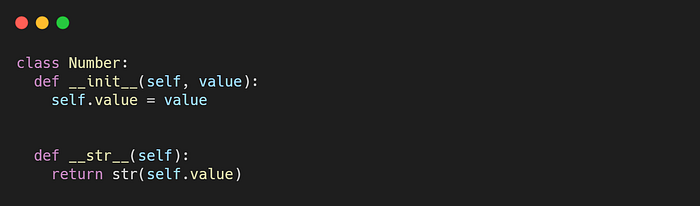We create a list of objects Number with values from 10 to 100 and then we print each element using the method `__str__()`.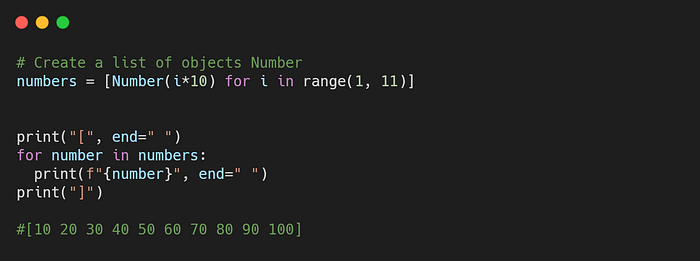Next, we create a new object called “num” with the value of 20 and check if the object exists in the list.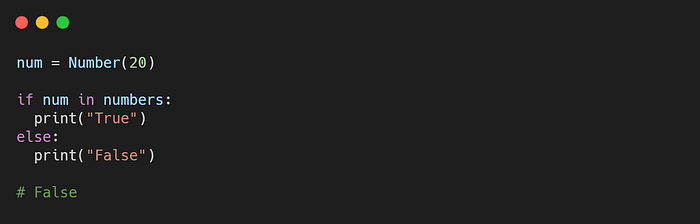But to our surprise, we get a False output, even if the number 20 exists in the list. This is because for the objects of the classes defined by the user we have to determine how they will be compared with each other. Python has no idea how to compare two objects of class Number.

At this point, Python reminds us how powerful it is. It has several super-powerful methods called “dunder methods” or “special methods” that give extra functionality to our classes.

To define how to compare two objects of our class, we need to implement the dunder method `__eq__()`. When we use the expression `a==b` Python invokes `A.__eq__(B)` since it exists. In our example, we implement this method as shown below: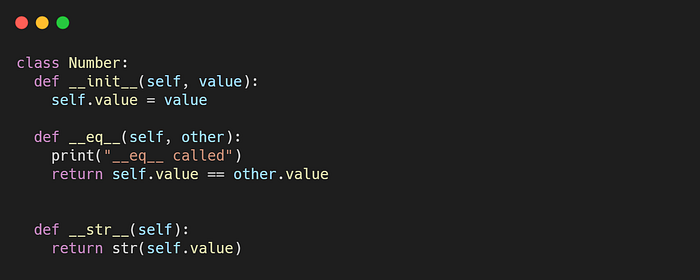In this way, we “tell” Python to compare the objects of our class depending on the attribute value.

So, if we execute the following code, the output will be True.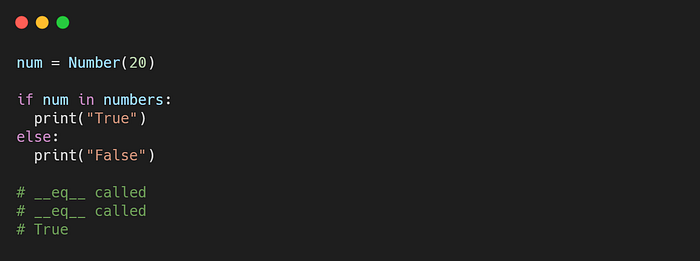We can see that the `__eq__()` method was called two times because value 20 is in index 1 of the list. Now, let's try another example. We define a variable num with the value 10 and check if the value exists in the list. But instead of True, the output is an AttributeError.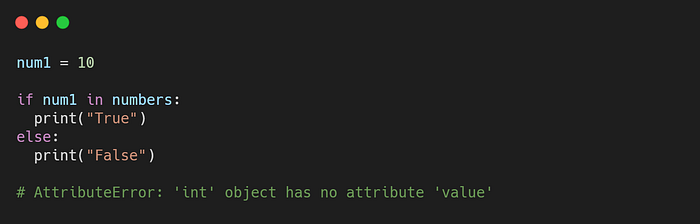That’s because we try to compare an int object with a Number object and as we defined the `__eq__()` the int object has no attribute value. So, to fix this, we will add a check in the `__eq__()` method as shown below: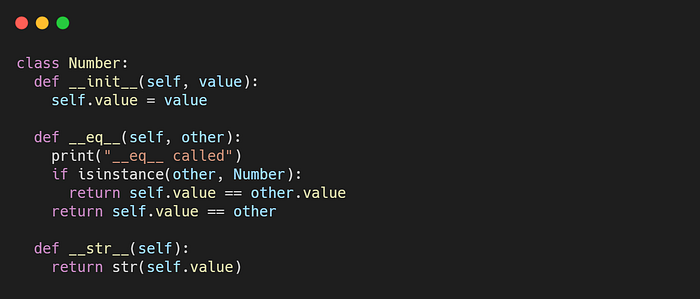Now, if the “other” object is an instance of class Number, we will check both objects by their attribute value. Otherwise, we will check the value attribute of the Number object with the value of the other.

Finally, we have the correct output.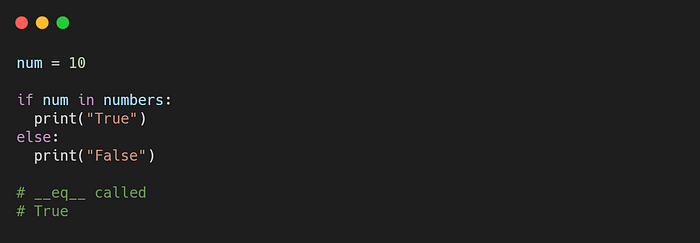In this tutorial, we saw the `__eq__()` dunder method. This method is important if we want to compare objects of our class with other entities. Moreover, we saw that we can define how to compare the object of our class with other objects depending on their class using the isinstance(obj, class) function. Remember that the under methods are really powerful, so it’s worth taking the time to study them. Till next time, keep learning and keep coding.